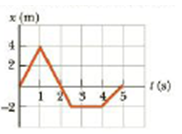Chapter 2, Problem 8P

Chapter
Section
Textbook Problem

A tennis player moves in a straight-line path as shown in Figure P2.8. Find her average velocity in the time intervals from (a) 0 to 1.0 s, (b) 0 to 4.0 s, (c) 1.0 s to 3.0 s, and (d) 0 to 5.0 s.Figure P2.8

(a)

To determine
The average velocity of the player in 0 to 1.0s .

Explanation

Given info: The initial position of the player in the interval is 0 , the final position of the player in the interval is 4.0m , the initial time of the interval is 0 , and the final time of the interval is 1.0s .

Explanation:

The formula used to calculate the average velocity is,

v¯=xfxitfti

v¯ is the average velocity.

xf is the final position.

xi s the initial position.

tf is the initial time.

ti is the final time.

Substitute 4.0m for xf , 0 for xi , 1

(b)

To determine
The average velocity of the player in 0 to 4.0s .

(c)

To determine
The average velocity of the player in 1.0s to 5.0s .

(d)

To determine
The average velocity of the player in 0 to 5.0s .

Still sussing out bartleby?

Check out a sample textbook solution.

See a sample solution

The Solution to Your Study Problems

Bartleby provides explanations to thousands of textbook problems written by our experts, many with advanced degrees!

Get Started